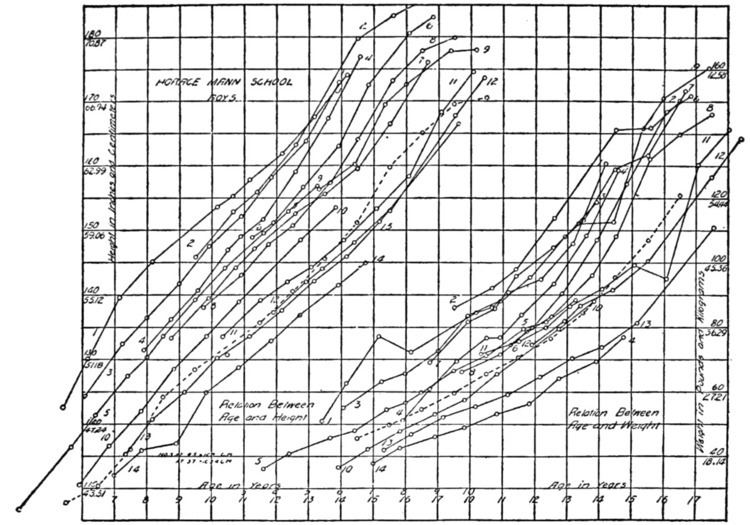# Growth curve (statistics)

Updated onThe growth curve model in statistics is a specific multivariate linear model, also known as GMANOVA (Generalized Multivariate ANalysis-Of-VAriance). It generalizes MANOVA by allowing post-matrices, as seen in the definition.

## Definition

Growth curve model: Let X be a p×n matrix, A a p×q matrix with q ≤ p, B a q×k matrix,C a k×n matrix with rank(C) + p ≤ n and let Σ be a positive-definite p×p matrix. Then

X = A B C + Σ 1 / 2 E

defines the growth curve model, where A and C are known, B and Σ are unknown, and E is a random matrix distributed as Np,n(0,Ip,n).

This differs from standard MANOVA by the addition of C, a "postmatrix".

## History

The growth curve model was invented by Potthoff and Roy in 1964; they used it to analyze repeated measurements of animals or humans to obtain a biological growth curve.

## Applications

GMANOVA is frequently used for the analysis of surveys, clinical trials, and agricultural data, as well as more recently in the context of Radar adaptive detection.

## Other uses

In mathematical statistics, growth curves such as those used in biology are often modeled as being continuous stochastic processes, e.g. as being sample paths that almost surely solve stochastic differential equations.

Similar Topics
Concealed 360
Steve Mungall
Robert Hague
Topics